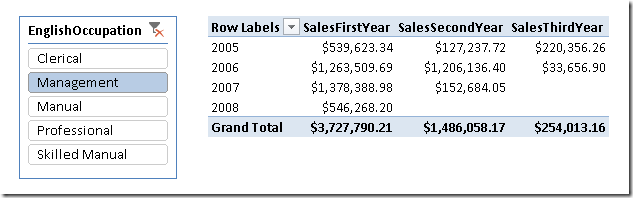I recently wrote an article that describes how to calculate a measure (such a sales, but it could be anything else) considering for each customer its first 12 months, then months 13-24 and so on. In practice, for every customer you have a different range of dates to consider, that starts on the date of its first order.In the past I implemented similar calculation in Analysis Services Multidimensional (MOLAP) by implementing a special dimension in the fact table, processed by the ETL, because otherwise the performance would have been very bad, not to mention the complexity of MDX involved. In Power Pivot and Tabular, however, it is much simpler and even if you can perform the calculation in a complete dynamic way, from a performance point of view it is better to leverage on a calculated column that persists the date of the first order for each customer. If you are curios of looking at the total dynamic approach, you can take a look at the DAX query below. The problem is that performance is 2 seconds by filtering only the Management occupation, and it becomes 13 seconds if you remove the Occupation filter at all. The solution described in the article (which include also sample Excel workbooks) simply moves the FirstOrder calculation in a calculated column, so the FILTER can be reduced iterating only the FirstOrder column and the performance is much better, with response time almost always in the 1-2 seconds area.

```DEFINE
MEASURE Customer[FirstOrder] =
CALCULATE (
MIN ( 'Internet Sales'[Order Date] ),
ALL ( 'Date' )
)
MEASURE Customer[SalesFirstYear] =
SUMX(
FILTER (
Customer,
CONTAINS ( 'Date', 'Date'[Date], [FirstOrder] )
),
CALCULATE (
SUM ( 'Internet Sales'[Sales Amount] ),
DATESINPERIOD (
'Date'[Date],
[FirstOrder],
12,
MONTH
)
)
)
MEASURE Customer[SalesSecondYear] =
SUMX(
FILTER (
Customer,
CONTAINS ( 'Date', 'Date'[Date], [FirstOrder] )
),
CALCULATE (
SUM ( 'Internet Sales'[Sales Amount] ),
DATESINPERIOD (
'Date'[Date],
[FirstOrder],
12,
MONTH
),
1,
YEAR
)
)
)
MEASURE Customer[SalesThirdYear] =
SUMX(
FILTER (
Customer,
CONTAINS ( 'Date', 'Date'[Date], [FirstOrder] )
),
CALCULATE (
SUM ( 'Internet Sales'[Sales Amount] ),
DATESINPERIOD (
'Date'[Date],
[FirstOrder],
12,
MONTH
),
2,
YEAR
)
)
)
EVALUATE
CALCULATETABLE (
SUMMARIZE (
'Internet Sales',
'Date'[Calendar Year]
),
"First Order", [FirstOrder],
"Sales 1st", [SalesFirstYear],
"Sales 2nd", [SalesSecondYear],
"Sales 3rd", [SalesThirdYear]
),
Customer[Occupation] = "Management"
)
```
FILTERReturns a table that has been filtered.

`FILTER ( <Table>, <FilterExpression> )`